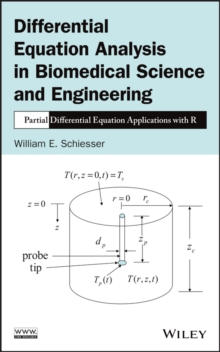Supporting your high street Find out how »
• My Account# Differential Equation Analysis in Biomedical Science and Engineering : Partial Differential Equation Applications with R Hardback

#### Description

Features a solid foundation of mathematical and computational tools to formulate and solve real-world PDE problems across various fields With a step-by-step approach to solving partial differential equations (PDEs), Differential Equation Analysis in Biomedical Science and Engineering: Partial Differential Equation Applications with R successfully applies computational techniques for solving real-world PDE problems that are found in a variety of fields, including chemistry, physics, biology, and physiology.

The book provides readers with the necessary knowledge to reproduce and extend the computed numerical solutions and is a valuable resource for dealing with a broad class of linear and nonlinear partial differential equations.

The author s primary focus is on models expressed as systems of PDEs, which generally result from including spatial effects so that the PDE dependent variables are functions of both space and time, unlike ordinary differential equation (ODE) systems that pertain to time only.

As such, the book emphasizes details of the numerical algorithms and how the solutions were computed. Featuring computer-based mathematical models for solving real-world problems in the biological and biomedical sciences and engineering, the book also includes: * R routines to facilitate the immediate use of computation for solving differential equation problems without having to first learn the basic concepts of numerical analysis and programming for PDEs * Models as systems of PDEs and associated initial and boundary conditions with explanations of the associated chemistry, physics, biology, and physiology * Numerical solutions of the presented model equations with a discussion of the important features of the solutions * Aspects of general PDE computation through various biomedical science and engineering applications Differential Equation Analysis in Biomedical Science and Engineering: Partial Differential Equation Applications with R is an excellent reference for researchers, scientists, clinicians, medical researchers, engineers, statisticians, epidemiologists, and pharmacokineticists who are interested in both clinical applications and interpretation of experimental data with mathematical models in order to efficiently solve the associated differential equations. The book is also useful as a textbook for graduate-level courses in mathematics, biomedical science and engineering, biology, biophysics, biochemistry, medicine, and engineering.

#### Information

• Format: Hardback
• Pages: 344 pages
• Publisher: John Wiley & Sons Inc
• Publication Date:
• Category: Biomedical engineering
• ISBN: 9781118705186

£89.95

£75.55£138.00

£131.10

£109.99

£109.50

£93.08

£40.00

£33.55